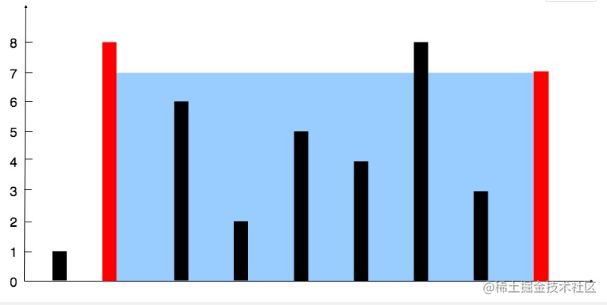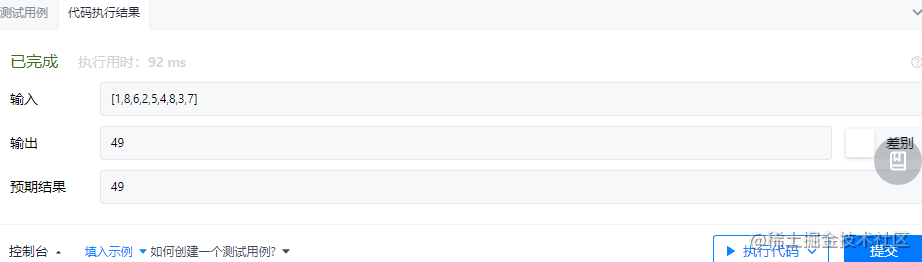## 一、题目

### 1、算法题目

“根据输入的数组数字构建坐标轴，求出坐标轴构成的容器可以容纳最多的水。”

### 2、题目描述``````输入：height = [1,8,6,2,5,4,8,3,7]

``````示例 2：

``````示例 3：

``````示例 4：

## 二、解题

### 1、思路分析

S(i,j) = min(h[i],h[j]) x (j-i)

### 2、代码实现

``````public class Solution
{
public int MaxArea(int[] height)
{
int l = 0, r = height.Length - 1, ans = -1, curr = -1;
while (l <= r) {
curr = (r - l) * Math.Min(height[l], height[r]);
ans = Math.Max(curr, ans);
if (height[l] < height[r]) l++;
else r--;
}
return ans;
}
}